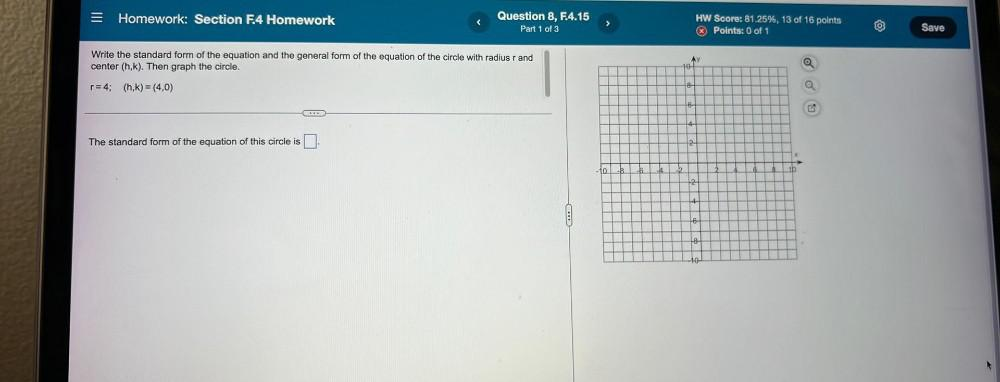Question:

# = Homework: Section F.4 Homework Question 8, F.4.15 Part 1 of 3 HW Score: 81.25%, 13 of 16 points Points: 0 of 1 Save Write the= Homework: Section F.4 Homework Question 8, F.4.15 Part 1 of 3 HW Score: 81.25%, 13 of 16 points Points: 0 of 1 Save Write the standard form of the equation and the general form of the equation of the circle with radius r and center (h,k). Then graph the circle. AY 10-1 @ r=4; (h,k) =(4,0) 8 O 6 4 The standard form of the equation of this circle is 2- - 10 . 1 2 4 10-